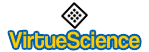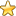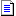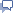Sponsored Question: Daily Deals on eBay or Daily Deals on Amazon??You are here: IndexThe Database of Number Correlations## Divisibility Rules For Prime Divisors

by Kalagara Venkata Lakshmi Narayana

Studying methods that can be used to determine whether a number is evenly divisible by other numbers, is an important topic in elementary number theory.

These are shortcuts for testing a number's factors without resorting to division calculations.

The rules transform a given number's divisibility by a divisor to a smaller number's divisibilty by the same divisor.

If the result is not obvious after applying it once, the rule should be applied again to the smaller number.

In childrens' math text books, we will usually find the divisibility rules for 2, 3, 4, 5, 6, 8, 9, 11.

Even finding the divisibility rule for 7, in those books is a rarity.

In this article, we present the divisibility rules for prime numbers in general and apply it to specific cases, for prime numbers, below 50.

We present the rules with examples, in a simple way, to follow, understand and apply.

Divisibility Rule for any prime divisor 'p' :

Consider multiples of 'p' till  (least multiple of 'p' + 1) is a multiple of 10, so that one tenth of (least multiple of 'p' + 1) is a natural number.

Let us say this natural number is 'n'.

Thus, n = one tenth of (least multiple of 'p' + 1).

Find  (p - n) also.

Example (i) :

Let the prime divisor be 7.

Multiples of 7 are 1x7, 2x7, 3x7, 4x7, 5x7, 6x7,

7x7 (Got it. 7x7 = 49 and 49+1=50 is a multiple of 10).

So 'n' for 7 is one tenth of (least multiple of 'p' + 1) = (1/10)50 = 5

'p-n' = 7 - 5 = 2.

Example (ii) :

Let the prime divisor be 13.

Multiples of 13 are 1x13, 2x13,

3x13 (Got it. 3x13 = 39 and 39+1=40 is a multiple of 10).

So 'n' for 13 is one tenth of (least multiple of 'p' + 1) = (1/10)40 = 4

'p-n' = 13 - 4 = 9.

The values of 'n' and 'p-n' for other prime numbers below 50 are given below.

p    n   p-n

7    5    2

13   4    9

17  12    5

19   2   17

23   7   16

29   3   26

31  28    3

37  26   11

41  37    4

43  13   30

47  33   14

After finding 'n' and 'p-n', the divisibility rule is as follows :

To find out, if a number is divisible by 'p', take the last digit of the number, multiply it by 'n', and add it to the rest of the number.

or multiply it by '(p - n)' and subtract it from the rest of the number.

If you get an answer divisible by 'p' (including zero), then the original number is divisible by 'p'.

If you don't know the new number's divisibility, you can apply the rule again.

So to form the rule, we have to choose either 'n' or 'p-n'.

Usually, we choose the lower of the two.

With this knlowledge, let us state the divisibilty rule for 7.

For 7, p-n (= 2) is lower than n (= 5).

Divisibility Rule for 7 :

To find out, if a number is divisible by 7, take the last digit, Multiply it by two, and subtract it from the rest of the number.

If you get an answer divisible by 7 (including zero), then the original number is divisible by 7.

If you don't know the new number's divisibility, you can apply the rule again.

Example 1 :

Find whether 49875 is divisible by 7 or not.

Solution :

To check whether 49875 is divisible by 7 :

Twice the last digit = 2 x 5 = 10; Rest of the number = 4987

Subtracting, 4987 - 10 = 4977

To check whether 4977 is divisible by 7 :

Twice the last digit = 2 x 7 = 14; Rest of the number = 497

Subtracting, 497 - 14 = 483

To check whether 483 is divisible by 7 :

Twice the last digit = 2 x 3 = 6; Rest of the number = 48

Subtracting, 48 - 6 = 42 is divisible by 7. ( 42 = 6 x 7 )

So, 49875 is divisible by 7. Ans.

Now, let us state the divisibilty rule for 13.

For 13, n (= 4) is lower than p-n (= 9).

Divisibility Rule for 13 :

To find out, if a number is divisible by 13, take the last digit, Multiply it with 4, and add it to the rest of the number.

If you get an answer divisible by 13 (including zero), then the original number is divisible by 13.

If you don't know the new number's divisibility, you can apply the rule again.

Example 2 :

Find whether 46371 is divisible by 13 or not.

Solution :

To check whether 46371 is divisible by 13 :

4 x last digit = 4 x 1 = 4; Rest of the number = 4637

Adding, 4637 + 4 = 4641

To check whether 4641 is divisible by 13 :

4 x last digit = 4 x 1 = 4; Rest of the number = 464

Adding, 464 + 4 = 468

To check whether 468 is divisible by 13 :

4 x last digit = 4 x 8 = 32; Rest of the number = 46

Adding, 46 + 32 = 78 is divisible by 13. ( 78 = 6 x 13 )

(if you want, you can apply the rule again, here. 4x8 + 7 = 39 = 3 x 13)

So, 46371 is divisible by 13. Ans.

Now let us state the divisibility rules for 19 and 31.

for 19, n = 2 is more convenient than (p - n) = 17.

So, the divisibility rule for 19 is as follows.

To find out, whether a number is divisible by 19, take the last digit, multiply it by 2, and add it to the rest of the number.

If you get an answer divisible by 19 (including zero), then the original number is divisible by 19.

If you don't know the new number's divisibility, you can apply the rule again.

For 31, (p - n) = 3 is more convenient than n = 28.

So, the divisibility rule for 31 is as follows.

To find out, whether a number is divisible by 31, take the last digit, multiply it by 3, and subtract it from the rest of the number.

If you get an answer divisible by 31 (including zero), then the original number is divisible by 31.

If you don't know the new number's divisibility, you can apply the rule again.

Like this, we can define the divisibility rule for any prime divisor.

The method of finding 'n' given above can be extended to prime numbers above 50 also.

Before, we close the article, let us see the proof of Divisibility Rule for 7

Proof of Divisibility Rule for 7 :

Let 'D' ( > 10 ) be the dividend.

Let D1 be the units' digit and D2 be the rest of the number of D.

i.e. D = D1 + 10D2

We have to prove

(i) if D2 - 2D1 is divisible by 7, then D is also divisible by 7

and (ii) if D is divisible by 7, then D2 - 2D1 is also divisible by 7.

Proof of (i) :

D2 - 2D1 is divisible by 7.

So, D2 - 2D1 = 7k where k is any natural number.

Multiplying both sides by 10, we get

10D2 - 20D1 = 70k

Adding D1 to both sides, we get

(10D2 + D1) - 20D1 = 70k + D1

or (10D2 + D1) = 70k + D1 + 20D1

or D = 70k + 21D1 = 7(10k + 3D1) = a multiple of 7.

So, D is divisible by 7. (proved.)

Proof of (ii) :

D is divisible by 7

So, D1 + 10D2 is divisible by 7

D1 + 10D2 = 7k where k is any natural number.

Subtracting 21D1 from both sides, we get

10D2 - 20D1 = 7k - 21D1

or 10(D2 - 2D1) = 7(k - 3D1)

or 10(D2 - 2D1) is divisible by 7

Since 10 is not divisible by 7, (D2 - 2D1) is divisible by 7. (proved.)

In a similar fashion, we can prove the divisibility rule for any prime divisor.

 For more about Divisibility Rules, go to, http://www.math-help-ace.com/Divisibility-Rules.html Name : KVLN Age : 47 years old Qualifications : B.Tech., M.S. (from IIT, Madras) Has 14 years of teaching experience. Loves math and chess. Winner of state rank in the mathematical olympiad. University level chess player. Love for math and love for teaching makes him feel more than happy to help. For First-Rate Math Help, go to the author's web site http://www.math-help-ace.com/It Aims to help to make every one an ace (expert) in math. Explains lucidly math topics for kids and teens with solved examples and exercises. Highlights the salient points and formulas. Helps to (i)develop confidence and desire to continue. (ii)perceive the work as less demanding. (iii)complete their math home work more quickly. (iii)perceive the work as less demanding. (iv)integrate the current problem with existing knowledge and ideas. (v)encourage them to reach a solution on their own, with their active mental participation. Helps every student succeed in math by making the journey a pleasant one.The topics you can learn and enjoy include basic algebra and other algebra topics such as Equations, Inequalities, Polynomials, Factoring, Exponents, Logarithms etc. The pleasant journey includes Number Systems and other Numbers topics such as Divisibilty Rules, Prime Factorization, G.C.F., L.C.M., Prime Numbers, Perfect Numbers, Whole Numbers, Integers, Fractions, Decimals, Rational Numbers, Irrational Numbers, Real Numbers etc. Basic operations on numbers such as addition and subtraction including a number of solved examples and Exercises, multiplication including Multiplication Tables and Division including Long Division are lucidly explained. Math Word problems on Addition, Subtraction, Multiplication, Division, G.C.F., L.C.M., Linear Equations in one and Two Variables, Quadratic Equations etc., are lucidly explained.

You are here: IndexThe Database of Number CorrelationsShare any properties and meanings for particular Numbers...contact me directly, thanks.Sponsored Question: Daily Deals on eBay or Daily Deals on Amazon??

Daily Deals on eBay

Hi, I am James Barton the founder of VirtueScience and Author of "Inner Medicine" which details my discoveries regarding the virtues along with practical exercises to awaken natural virtue. I have a wide range of interests but the main focus of this site now is the virtues and character. Please join free to contribute to the blogs and forums.